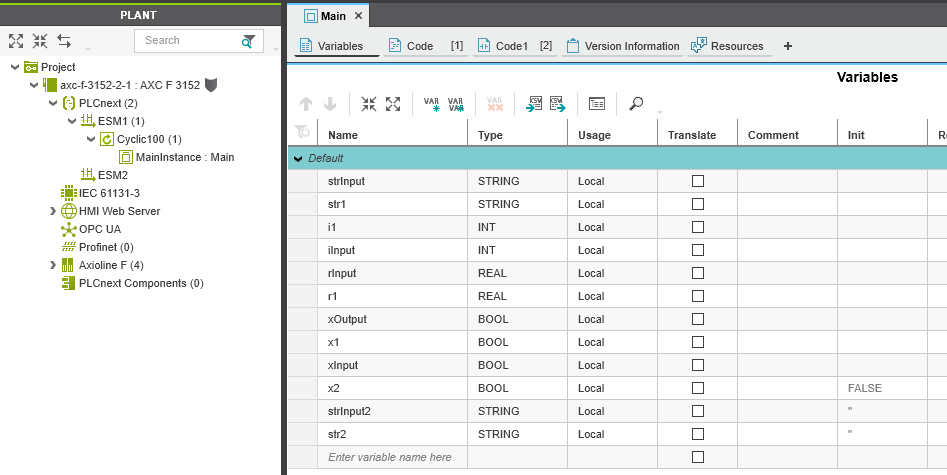Makers Blog API gRPC PLCnext Technology Python

# gRPC Python – Read and Write Process Data

nilshettig 12 August 2022

This article describes how to access and write simple process data with Python with an AXC F 3152 utilizing gRPC. (https://www.plcnext.help/te/Service_Components/gRPC_Introduction.htm)

### Prerequisite

First we have to prepare the required files, outside the PLC, e.g., on a Windows machine.

1. Install Python 3.9 (3.10 may cause errors)
2. Install the required Python package to generate code from the .proto files: `pip install grpcio-tools==1.36.1`
3. Download and unzip the repository containing the .proto files from https://github.com/PLCnext/gRPC

## Generate _pb2.py and _pb2_grpc.py from .proto Files

Next, we have to generate the required python files from the provided .proto files. The latter are located in the following folder: gRPC-master/protobuf.

Use this code to create a Python script in the Folder gRPC-master, e.g., generate_grpc.py. The script

1. generates the required files and place them in gRPC-master/pxc_grpc
``````
import glob
import os
from pathlib import Path

# create the output directory
Path('pxc_grpc').mkdir(parents=True, exist_ok=True)

grpc_command_base = 'python -m grpc_tools.protoc -I./protobuf --python_out=pxc_grpc --grpc_python_out=pxc_grpc '

import_paths = set()

# generate the *_pb2.py and *_pb2_grpc.py files
for filename in glob.iglob('./protobuf/**', recursive=True):

if filename.endswith('.proto'):

# store the import path
path_parts = filename.split(os.sep)

grpc_command = ''.join([grpc_command_base, os.path.join('.', os.path.relpath(filename))])
stream = os.popen(grpc_command)
if output != '':
print(''.join(['error/info for file ', os.path.relpath(filename), ' - ', output]))

# get the python files in the base directory
base_pys = set()

for (dirpath, dirnames, filenames) in os.walk('./pxc_grpc'):
for f in filenames:
break

# reformat the stored paths to adapt the import statements
try:
import_paths.remove('')
except:
pass

import_paths = list(import_paths)
import_paths.sort(key=len)
import_paths.reverse()

for filename in glob.iglob('./pxc_grpc/**', recursive=True):

if filename.endswith('.py'):

new_lines = []

with open(filename, 'r') as file:
for line in lines:
if line.startswith('from'):
for import_path in import_paths:
if import_path in line:
line = line.replace(import_path, ''.join(['pxc_grpc.', import_path]), 1)
break
elif line.startswith('import'):
parts = line.split()
if parts in base_pys:
line = line.replace('import', 'from pxc_grpc import')

new_lines.append(line)

with open(filename, 'w') as file:
file.write(''.join(new_lines))

``````

Open a shell and execute the script: `pyton generate_grpc.py`

## Create a PLCnext Demo Project

The shown project should only demonstrate how the gRPC interface interacts with the GDS. Feel free to use an existing project instead. For an individual project, you have to edit the port names in the following Python script accordingly, e.g., `Arp.Plc.Eclr/MainInstance.strInput`.## Prepare the PLC

Install pip to manage your Python packages:

1. Connect the AXC F 3152 controller to the Internet.
2. Enter the command curl https://bootstrap.pypa.io/get-pip.py -o get-pip.py.
3. Then enter the command python3 get-pip.py.

Install the required packages: `pip install grpcio protobuf==3.20.0`

Create a folder 'grpc_test' in the projects directory (/opt/plcnext/projects/).

Copy the 'pxc_grpc' folder, containing the crated Python files to 'grpc_test', e.g., with WinSCP.

Create a Python script in the 'grpc_test' folder named 'grpc_test.py' and insert the following code:

``````
import grpc
from pxc_grpc.Plc.Gds.IDataAccessService_pb2_grpc import IDataAccessServiceStub
from pxc_grpc.Plc.Gds.WriteItem_pb2 import WriteItem

def write_single_string(stub, port_name, value):

single_write_request = IDataAccessServiceWriteSingleRequest()
single_write_request.data.PortName = port_name
single_write_request.data.Value.TypeCode = 19
single_write_request.data.Value.StringValue = value

return stub.WriteSingle(single_write_request)

def write_single_int(stub, port_name, value):

single_write_request = IDataAccessServiceWriteSingleRequest()
single_write_request.data.PortName = port_name
single_write_request.data.Value.TypeCode = 6
single_write_request.data.Value.Int16Value = value

return stub.WriteSingle(single_write_request)

def write_multiple_values(stub):

write_request = IDataAccessServiceWriteRequest()

wi1 = WriteItem()
wi1.PortName = 'Arp.Plc.Eclr/MainInstance.strInput'
wi1.Value.StringValue = "test1"
wi1.Value.TypeCode = 19

wi2 = WriteItem()
wi2.PortName = 'Arp.Plc.Eclr/MainInstance.strInput2'
wi2.Value.StringValue = "test2"
wi2.Value.TypeCode = 19

# add multiple WriteItems at once
write_request.data.extend([wi1, wi2])

# response1.data.append(wi1)
# response1.data.append(wi2)

return stub.Write(write_request)

if __name__ == "__main__":

# create channel and stub
channel = grpc.insecure_channel('unix:/run/plcnext/grpc.sock')
stub = IDataAccessServiceStub(channel)

print(write_single_string(stub, 'Arp.Plc.Eclr/MainInstance.strInput', 'test123'))
print(write_single_int(stub, 'Arp.Plc.Eclr/MainInstance.iInput', 18))

print(write_multiple_values(stub))

print(r)
print(r._ReturnValue.Value.TypeCode)
print(r._ReturnValue.Value.StringValue)

for value in r._ReturnValue:
print(value, value.Value.TypeCode)

``````

## Run the Example

Now start the example. Login on the PLC via ssh and navigate to 'grpc_test', then start the Python script:

1. `cd projects/grpc_test/`
2. `python3 grpc_test.py`

The gRPC enables interaction with GDS variables.

## Data Types

To read and write variables, the data type is required, e.g., `wi1.Value.TypeCode = 19`. The types are described in the genereated file `gRPC-master/pxc_grpc/ArpTypes_pb2.py` starting in line 242:

``````CT_None = 0
CT_End = 0
CT_Void = 1
CT_Boolean = 2
CT_Char = 3
CT_Int8 = 4
CT_Uint8 = 5
CT_Int16 = 6
CT_Uint16 = 7
CT_Int32 = 8
CT_Uint32 = 9
CT_Int64 = 10
CT_Uint64 = 11
CT_Real32 = 12
CT_Real64 = 13
CT_Struct = 18
CT_String = 19
CT_Utf8String = 19
CT_Array = 20
CT_DateTime = 23
CT_Version = 24
CT_Guid = 25
CT_AnsiString = 26
CT_Object = 28
CT_Utf16String = 30
CT_Stream = 34
CT_Enumerator = 35
CT_SecureString = 36
CT_Enum = 37
CT_Dictionary = 38
CT_SecurityToken = 39
CT_Exception = 40
CT_IecTime = 41
CT_IecTime64 = 42
CT_IecDate = 43
CT_IecDate64 = 44
CT_IecDateTime = 45
CT_IecDateTime64 = 46
CT_IecTimeOfDay = 47
CT_IecTimeOfDay64 = 48
``````

The corresponding value variables, e.g., `r._ReturnValue.Value.StringValue`, could be found in the same file, beginning in line 365, e.g., `BoolValue`, `Int8Value`, `StringValue`.

### Discussionb.kuipers 25.11.2022

Hello, I was trying to run this example, but I recieved the following error when trying to run the grpc_test.py scripy on the PLC: ImportError: cannot import name 'cygrpc' from 'grpc._cython' (/opt/plcnext/.local/lib/python3.10/site-packages/grpc/_cython/__init__.py) Any idea what this could be?